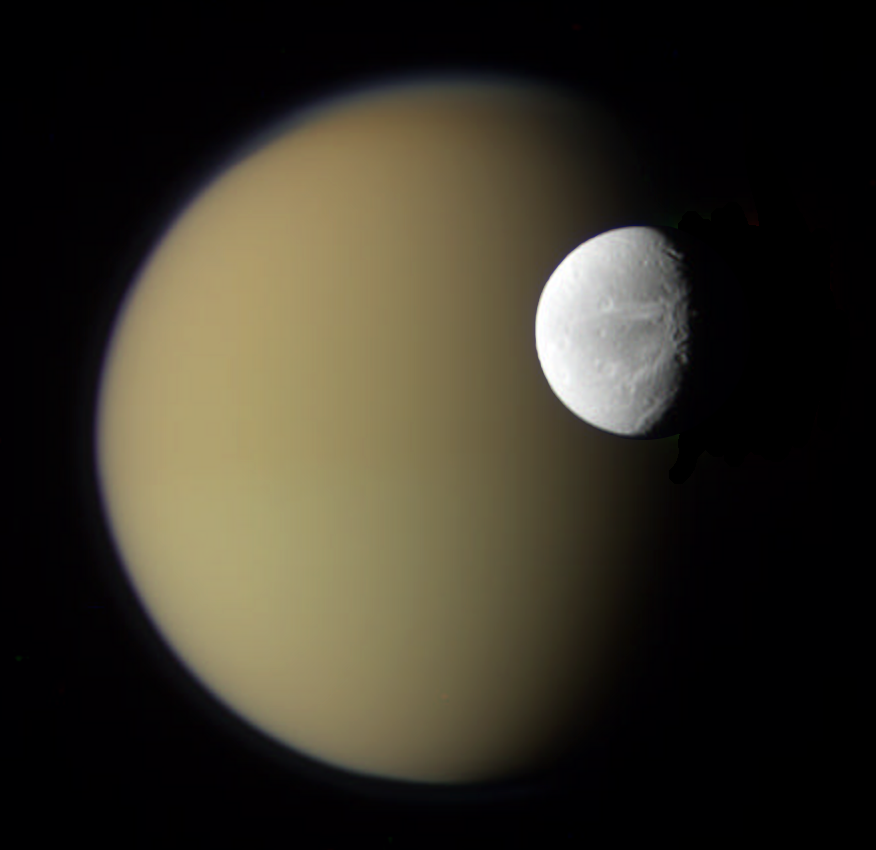SPOILERS FOR INFINITY WAR! Though it is seen in the trailers and promotional materials.
Spoiler:Lot's of work and some spoilers, so let's get to work.
Spoiler:
After a bit of research, the closest moon to Titan is Dione.
https://apod.nasa.gov/apod/ap100420.html
https://www.nasa.gov/mission_pages/cassini/multimedia/pia14910.html
Spoiler:Dione has an average density of 1480kg/m^3 and a total mass of 10^20kg (1.0e20kg) and has a diameter of 1123km.
Spoiler:object degree size = 2*atan(Object_Size/(Panel_Height/tan(70/2)))
= 2*atan(401/(1080/tan(70/2)))
= 19.955394639887441 degrees

Enter that through the angscaler and we get 3191.7km away. Let's get the timeframe for the initial fall.
Spoiler:3.06 seconds.

T = 3191.7km/3.06s
= 1043039.22/340.29
= Mach 3065.14802

Spiderman reacts to the meteorites and saves Mantis and Drax from them, so it scales to their reactions (and it's consistent with Yondu exscaping Ego and Thor's hammer reaching the Moon). Now, onto the energy to rip apart the moons surface and drag it down.
Spoiler:R = (h/2) + c^2/(8h)
= (13/2) + 295^2/(8 X 13)
= 843.278846

843.278846 X 2 = 1686.55769 pixels
1686.55769 pixels = 1123km
1 pixel = 1123km/1686.55769 = 0.665853298km
0.665853298km X 4 = 2.66341319km
1123km/2 = 561.5km (561500m)
561.5km - 2.66341319km = 558.836587km (558836.587m)

V = 4/3πr^3
= 4/3 X π X 558836.587^3
= 7.31043305e17m^3

V = 7.41545649e17 - 7.31043305e17
= 1.0502344e16m^3

It seems to be mainly the front side, so we'll half that area.

V = 1.0502344e16/2
= 5.251172e15m^3

Violent fragmentation of rock is 69000000 joules/m^3.

E = 1.3826457e16 X 69000000
= 9.54025533e23 joules

We'll add that on to the KE at the end.

M = 5.251172e15 X 1480
= 7.77173456e18kg

KE = (0.5) X 7.77173456e18 X 964259.819^2
= 3.61306773e30 joules

A friend point out in the fight, that not only was a continent ripped apart, but the entire moon itself.
2:48
Spoiler:So the entire moon was fragmented and thrown it seems. We'll go with a high end of that in anycase.

V = 4/3πr^3
= 4/3 X π X 561500^3
= 7.41545649e17m^3

Violent fragmentation of rock is 69000000 joules/m^3.

E = 7.41545649e17 X 69000000
= 5.11666498e25 joules

Let's also get the KE.

KE = (0.5) X 1.0e20 X 964259.819^2
= 4.64898499e31 joules
= 11.1113407983 zettatons

Final Results
Meteorites fall to Titan = Mach 3065.148
Meteroite hits Iron Man = 2.126 gigatons
Thanos rips moons surface off (low end) = 863.544 exatons
Thanos rips moons surface off (high end) = 11.111 zettatons

I may have another look when a better quality version is released, but damn if that's not impressive.